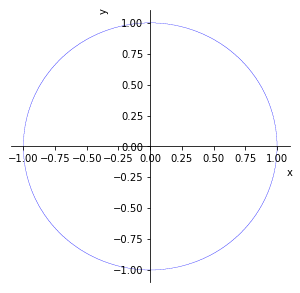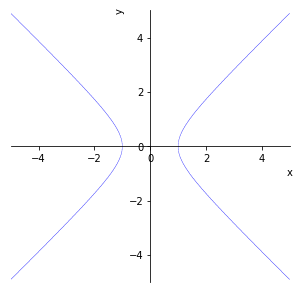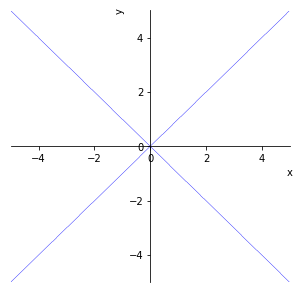Create a graph with Sympy

When doing algebraic calculations using Sympy, you may want to take a quick look at what the graph of this formula looks like. Speaking of graphs in Python, I think the first thing that comes to mind is matplotlib, but when \$ y = f (x) \$, \$ x \$ needs to be assigned using numpy's arrange, which is troublesome. .. Sympy's Plotting module can be used in such cases. This not only graphs the formula as it is, but also does something quite complicated.

Import required modules

First, import the required modules. In Sympy, it seems that "from sympy import ~" is often used. In addition to the var that defines the variables, this time I will use the "plot_implicit function" that allows you to experience the greatness of the Plotting module. Also, in fact, the graph of Sympy has matplotlib running behind the scenes, so import this as well.

Import necessary modules etc.

from sympy import var
from sympy.plotting import plot_implicit
import matplotlib.pyplot as plt

Creating a circle

First, let's draw a circle (\$ x ^ 2 + y ^ 2 = 1 \$).

Import necessary modules etc.

#Drawing an ellipse
var('x,y')
size = 1.1
f = x**2 + y**2 - 1
plot_implicit(f, (x, -size, size), (y, -size, size),
xlim=(-size*1.1, size*1.1), ylim=(-size*1.1, size*1.1))
plt.rcParams['figure.figsize'] = (5, 5)

With plot_implicit, you can graph the formula nicely without having to make it look like \$ y = f (x) \$. You can create a graph just by entering a formula and specifying the range of \$ x, y \$ with plot_implicit. After that, in plt.rcParams of matplotloib, specify the size of the graph etc. and it is completed. The result is as follows:Drawing a hyperbola

Next, let's draw a hyperbola. The formula is \$ x ^ 2- y ^ 2 = 1 \$. The program and graph are as follows.

Import necessary modules etc.

#Drawing a hyperbola
var('x,y')
size = 5
f= x**2 - y**2 - 1
plot_implicit(f, (x, -size, size), (y, -size, size),
xlim=(-size*1.1, size*1.1), ylim=(-size*1.1, size*1.1))
plt.rcParams['figure.figsize'] = (5, 5)Two straight lines that intersect

Let's draw the last two straight lines that intersect. The formula is \$ f = x ^ 2 --y ^ 2 --0 \$. The program and graph are as follows.

Import necessary modules etc.

#Drawing intersecting curves
var('x,y')
size = 5
f= x**2 - y**2 - 0
plot_implicit(f, (x, -size, size), (y, -size, size),
xlim=(-size*1.1, size*1.1), ylim=(-size*1.1, size*1.1))
plt.rcParams['figure.figsize'] = (5, 5)Summary

Suddenly I used a special function, but I think it's very convenient. However, it seems that plot_implicit cannot be used in 3D, so you need to use another module. I'm doing a little more research on this.